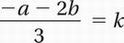# SAT Math Multiple Choice Question 763: Answer and Explanation

### Test Information

Question: 763

13.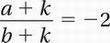Given the formula above, which of the following expresses k in terms of a and b?

• A.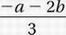• B.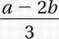• C.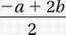• D.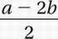Explanation:

A

Algebra (linear equations) MEDIUM

Original equation: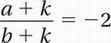Multiply by b + k:

a + k = -2(b + k)

Distribute:

a + k = -2b - 2k

Subtract k:

a = -2b - 3k